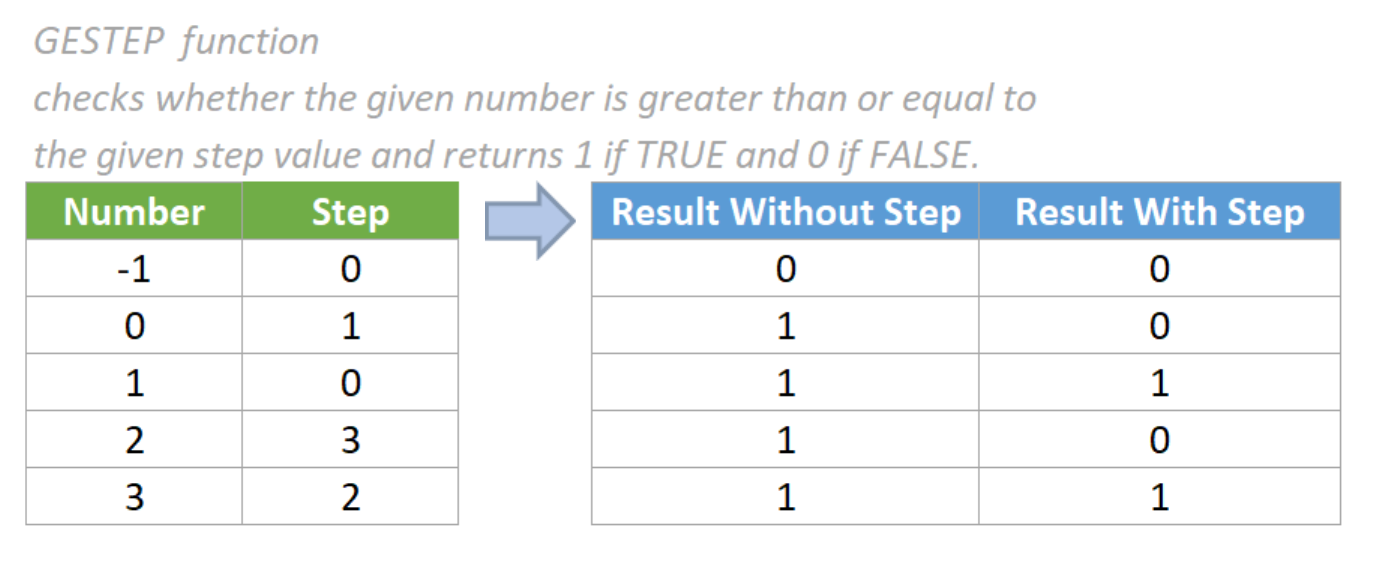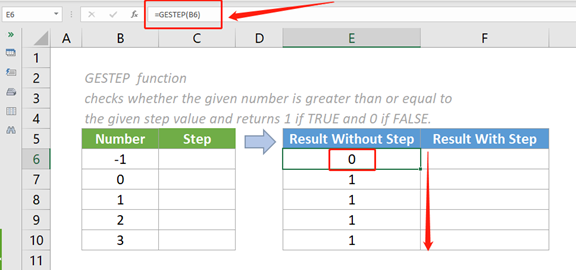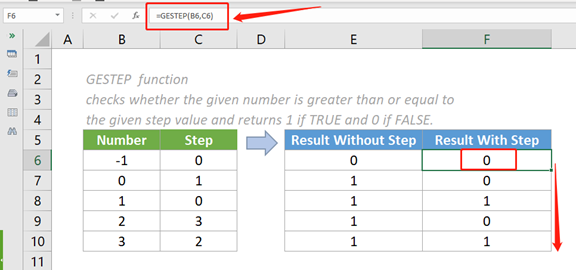## Excel GESTEP Function

The GESTEP function checks whether the given number is greater than or equal to the given step value and returns 1 if TRUE and 0 if FALSE.#### Syntax

=GESTEP (number, [step])

#### Arguments

• Number (required): The number to be tested.
• Step (optional): The threshold value that the supplied number is to be compared to. If omitted, it will default to zero (0).

#### Return value

The GESTEP function returns a numeric value.

#### Function notes

1. The GESTEP function is available in Excel 2007 and later Excel Versions.
2. The GESTEP function can be used to filter a set of values. For example, by summing the returned results from the GESTEP function we can calculate the count of values that exceed a threshold.
3. For users who are familiar with the IF function, the below syntax can achieve the same goal as the GESTEP function.
Even though the GESTEP function is easier to use, it is not known by many Excel users, therefore I highly recommend it here.

=IF (number 1>= number 2,1, 0)

4. The #VALUE! error value occurs if any of the supplied arguments are non-numeric.

#### Examples

##### Example One: Result Without Step

In this case, we want to compare the number in the table below with the value zero and return 1 if the number ≥ 0 and 0 if the number < 0. Please do as follows.

1. Please copy the formula below into cell E6, then press the Enter key to get the result.

=GESTEP (B6)

2.Select this result cell and drag its autofill handle down to get the rest of the results.Notes:

1. The step argument is omitted in the above formula. Therefore, the default value of the step argument is 0.
2. The argument in the above formulas is supplied as a cell reference containing a numeric value.
3. We can also directly input a value in the formula. For example, the formula in cell E6 can be changed to:

=GESTEP (-1)

##### Example Two: Result with Step

In this case, we want to compare the number with the step value in the table below and return 1 if the number ≥ step and 0 if the number < step. Please do as follows.

1. Please copy the formula below into cell F6, then press the Enter key to get the result.

=GESTEP (B6, C6)

2.Select this result cell and drag its autofill handle down to get the rest of the results.Notes:

1. The arguments in the above formula are supplied as cell references containing numeric values.
2. We can also directly input values in the formula. For example, the formula in cell F6 can be changed to:

=GESTEP (-1, 0)

#### Relative Functions:

• Excel EVEN Function
The EVEN function rounds numbers away from zero to the nearest even integer.

• Excel EXP Function
The EXP function returns the result of the constant e raised to the nth power.

### The Best Office Productivity Tools

#### Kutools for Excel - Helps You To Stand Out From Crowd

 Popular Features: Find, Highlight or Identify Duplicates  |  Delete Blank Rows  |  Combine Columns or Cells without Losing Data  |  Round without Formula ... Super VLookup: Multiple Criteria  |  Multiple Value  |  Across Multi-Sheets  |  Fuzzy Lookup... Adv. Drop-down List: Easy Drop Down List  |  Dependent Drop Down List  |  Multi-select Drop Down List... Column Manager: Add a Specific Number of Columns  |  Move Columns  |  Toggle Visibility Status of Hidden Columns  |  Compare Columns to Select Same & Different Cells ... Featured Features: Grid Focus  |  Design View  |  Big Formula Bar  |  Workbook & Sheet Manager | Resource Library (Auto Text)  |  Date Picker  |  Combine Worksheets  |  Encrypt/Decrypt Cells  |  Send Emails by List  |  Super Filter  |  Special Filter (filter bold/italic/strikethrough...) ... Top 15 Toolsets:  12 Text Tools (Add Text, Remove Characters ...)  |  50+ Chart Types (Gantt Chart ...)  |  40+ Practical Formulas (Calculate age based on birthday ...)  |  19 Insertion Tools (Insert QR Code, Insert Picture from Path ...)  |  12 Conversion Tools (Numbers to Words, Currency Conversion ...)  |  7 Merge & Split Tools (Advanced Combine Rows, Split Excel Cells ...)  |  ... and more

Kutools for Excel Boasts Over 300 Features, Ensuring That What You Need is Just A Click Away...#### Office Tab - Enable Tabbed Reading and Editing in Microsoft Office (include Excel)

• One second to switch between dozens of open documents!
• Reduce hundreds of mouse clicks for you every day, say goodbye to mouse hand.
• Increases your productivity by 50% when viewing and editing multiple documents.
• Brings Efficient Tabs to Office (include Excel), Just Like Chrome, Edge and Firefox.